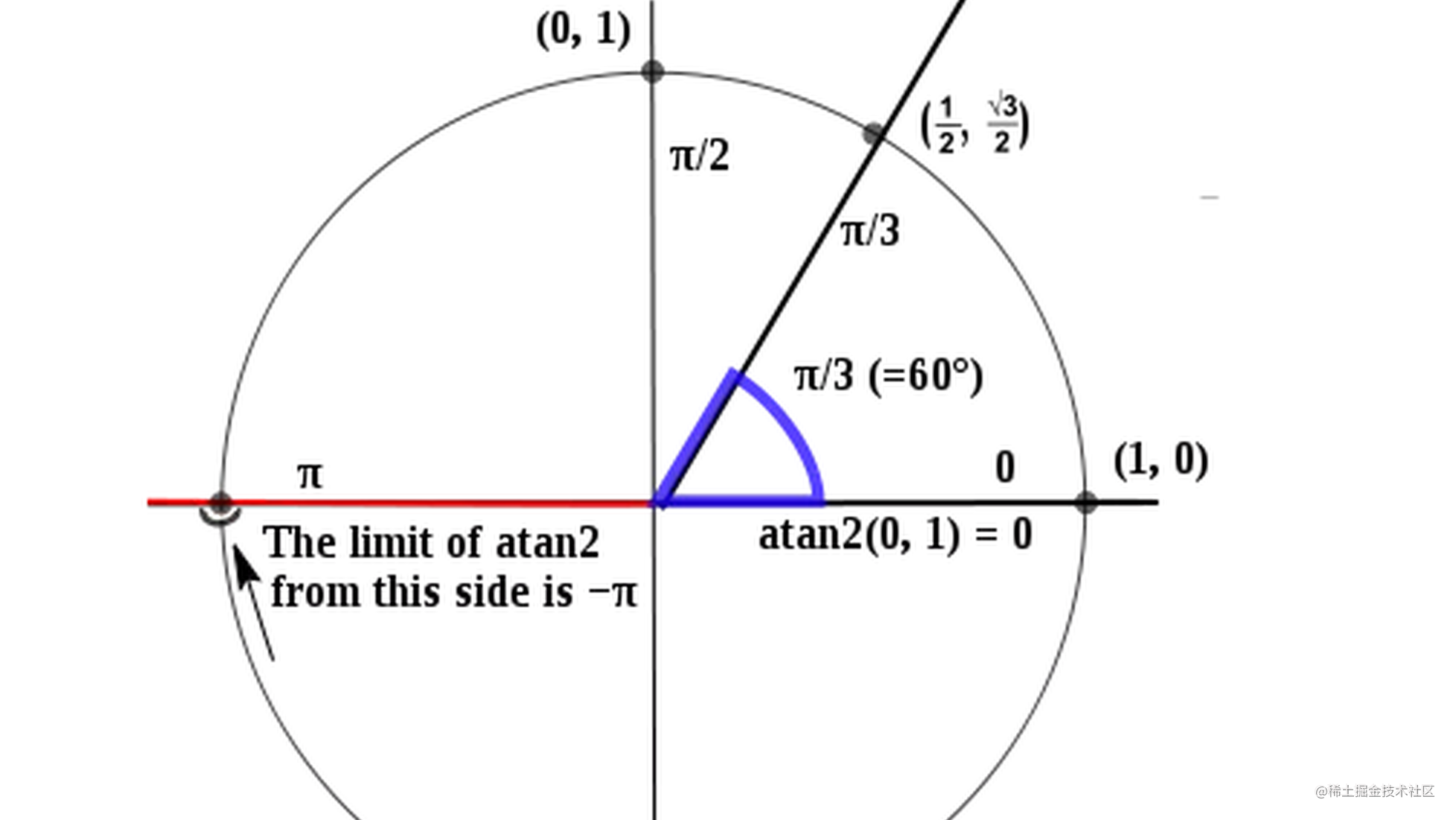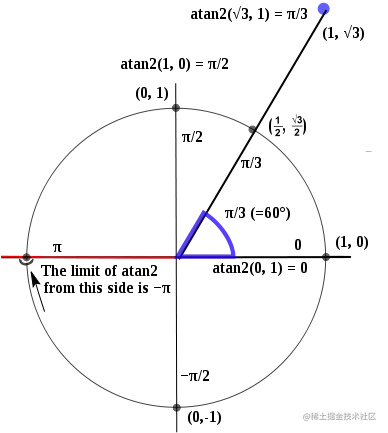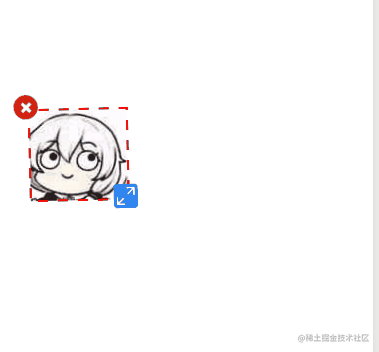# 更新记录

• 更新了Vue版本，并且将Vue版本发布到线上了

# 实现思路

## 实现图片的上传与存储

``````//index.js
const this1 = this
//小程序上传图片的api
wx.chooseImage({
count: 1,
sizeType: ["original", "compressed"],
sourceType: ["album"],
success(res) {
const tempFilePaths = res.tempFilePaths
//小程序获取图片信息的api
wx.getImageInfo({
src: tempFilePaths,
success(res) {
const {width,height} = res
const scale = this1.getScale(width,height) //如果图片宽高太大会缩放，实现的思路有很多
const obj = {
width:width/scale,
height:height/scale,
url: tempFilePaths
}
const imgArr = this1.data.imgArr
imgArr.push(obj)
this1.setData({
imgArr
})
}
})
}
})
}

## 获取canvas对象

``````//canvas-drag.js
this.data.ctx = wx.createCanvasContext("canvas",this);
},

## 定义图片的类

``````class dragImg {
constructor(img,ctx){
//x y为初始坐标
this.x = 100;
this.y = 100;
//w,h为初始宽高
this.w = img.width;
this.h = img.height;
this.url = img.url;
this.ctx = ctx;
this.rotate = 0;
this.selected = true;
}
}

## 接收并监听外部数组，并在canvas中绘制图片

### api的介绍与类方法的定义

• `ctx.drawImage` 描述或描绘图片到canvas。注意：小程序版本中先描述再描绘，非小程序版是直接描绘到画布上
• `ctx.draw` 将之前在绘图上下文中的描述（路径、变形、样式）画到 canvas 中。 注意：只在有小程序版本有此api
• `ctx.clearRect` 清除指定区域内容 用在非小程序版重绘画布

``````  paint() {
//计算图片中心的坐标，后续要用上
this.centerX = this.x + this.w / 2;
this.centerY = this.y + this.h / 2;
// 描述图片
this.ctx.drawImage(this.url, this.x, this.y, this.w, this.h);
// 如果是选中状态，绘制选择虚线框，和缩放图标、删除图标
if (this.selected) {
//对于canvas其他的描述api，因为不是重点就不详细描述出来，相信你们聪明得脑袋动动小手百度一下就懂了
this.ctx.setLineDash([10, 10]);
this.ctx.setLineWidth(2);
this.ctx.setStrokeStyle("red");
this.ctx.lineDashOffset = 10;
this.ctx.strokeRect(this.x, this.y, this.w, this.h);
this.ctx.drawImage(CloseIcon, this.x - 15, this.y - 15, 24, 24);
this.ctx.drawImage(ScaleIcon, this.x + this.w - 15, this.y + this.h - 15, 24, 24);
}
}

### 小程序版本绘制与重绘

``````//canvas-drag.js
properties: {
imgArr: {
type: Array,
value: [],
observer: "onArrChange"
},
},
onArrChange(arr){
if(arr.length){
//取到新的图片item
const newImg = arr.slice(-1)
const item = new dragImg(newImg, this.data.ctx)
this.data.dragArr.push(item)
this.draw()
}
},
draw(){
this.data.dragArr.forEach((item) => {
item.paint()
})
this.data.ctx.draw()
}

### 非小程序版本绘制与重绘

``````draw () {
//清空画板
this.ctx.clearRect(0, 0, this.c.width, this.c.height)
this.dragArr.forEach((item) => {
item.paint()
})
//由于ctx.draw是小程序独有的所以这里不再调用
},

## 点击定位(初版)

### 新的方法isInWhere

``````isInWhere(x, y) {

// 变换区域左上角的坐标和区域的高度宽度
let transformW = 24;
let transformH = 24;
let transformX = this.x + this.w ;
let transformY = this.y + this.h ;
// 删除区域左上角的坐标和区域的高度宽度
let delW = 24;
let delH = 24;
let delX = this.x ;
let delY = this.y ;
移动区域的坐标
let moveX = this.x;
let moveY = this.y;
if (x - transformX >= 0 && y - transformY >= 0 && transformX + transformW - x >= 0 && transformY + transformH - y >= 0) {
// 缩放区域
return "transform";
}
else if (x - delX >= 0 && y - delY >= 0 && delX + delW - x >= 0 && delY + delH - y >= 0) {
// 删除区域
return "del";
}
else if (x - moveX >= 0 && y - moveY >= 0 && moveX + this.w - x >= 0 && moveY + this.h - y >= 0) {
// 移动区域
return "move";
}
// 不在选择区域里面
return false;
}

### 在点击时调用

``````start(e){
const {x,y} = e.touches
this.data.dragArr.forEach((item)=>{
const place = item.isInWhere(x,y)
})
}

`console.log(place)`打印结果看看效果

## 多个图层下的点击

``````   start(e) {
//初始化一个数组用于存放所有被点击到的图片对象
this.data.clickedkArr = []
const { x, y } = e.touches
this.data.dragArr.forEach((item) => {
const place = item.isInWhere(x, y)
item.place = place
//先将所有的item的selected变为flase
item.selected = false
if (place==='move'&&'transform') {
//如果place不是false或者del就push进这个数组中
this.data.clickedkArr.push(item)
}
})
const length = this.data.clickedkArr.length
if (length) {
//我们知道cavans绘制的图片的层级是越来越高的，因此我们取这个数组的最后一项，保证取到的图片实例是层级最高的
const lastImg = this.data.clickedkArr[length - 1]
//将该实例的被选值设为true，下次重新绘制将绘制边框
lastImg.selected = true
//保存这个选中的实例
this.data.lastImg = lastImg
//保存这个实例的初始值，以后会用上
this.data.initialXY = {
initialX: lastImg.x,
initialY: lastImg.y,
initialH:lastImg.h,
initialW:lastImg.w,
initialRotate:lastImg.rotate
}
}
//重新绘制
this.draw()
//保存点击的坐标，move时要用
this.data.startTouch = { startX : x, startY : y }
},

## 实现平移效果

``````move(e) {
const { x, y } = e.touches
const { initialX, initialY } = this.data.initialXY
const { startX, startY } = this.data.startTouch
const lastImg = this.data.lastImg
if (this.data.clickedkArr.length) {
if (palce) {
//算出移动后的xy坐标与点击时xy坐标的差（即平移量）与图片对象的初始坐标相加即可
lastImg.x = initialX + (x - startX)
lastImg.y = initialY + (y - startY)
}
//transform后续将补全
if (this.data.lastImg.place === 'transform') {
}
this.draw()
}
}

## 实现旋转效果

• `Math.atan2` 用于计算手指滑动时移动的角度
• `ctx.rotate` 用于旋转canvas

### 利用Math.atan2计算旋转角度可以看到atan2( √3/2 , 1/2 )=π / 3,即坐标( 1/2 , √3/2 )的点与原点( 0 , 0 )确立出的直线与x轴形成的倾斜角为60度，所以原式又可以写成这样atan2( √3/2 - 0 , 1/2 - 0 ) = π / 3。

``````    //接上段move中的transform
if (this.data.lastImg.place === 'transform'){
const { centerX, centerY }= lastImg
const { initialRotate } = this.data.initial
//算出手指按下时形成的角度，注意y坐标在第一个参数
const angleBefore = Math.atan2(startY - centerY, startX - centerX) / Math.PI * 180;
//算出手指移动时形成的角度，注意y坐标在第一个参数
const angleAfter = Math.atan2(y - centerY, x - centerX) / Math.PI * 180;
// 旋转的角度
lastImg.rotate = initialRotate + angleAfter - angleBefore;
}
this.draw()

### 利用ctx.rotate旋转图片

`ctx.rotate`是用于旋转画布的坐标轴并非是旋转里面某个图片，以画布的原点，就是左上角(0,0)进行旋转(当然这个原点是可以更改的),我们在新的坐标轴上描述图片，因此就看起来就像是图片被旋转了一样。因此我们要将旋转点变更至图片的中点，这时又涉及另一个api了，那就是`ctx.translate()`。顾名思义，就是用来平移画布原点的方法。我们将画布的原点平移至图片的中点，旋转坐标轴后，又平移回来(因为后续描述图片还是得以(0,0)为原点。

``````  paint() {
this.ctx.save();
this.centerX = this.x + this.w / 2;
this.centerY = this.y + this.h / 2;
// 变更原点至图片的中点
this.ctx.translate(this.centerX, this.centerY);
//根据transform的旋转角度旋转坐标轴
this.ctx.rotate(this.rotate * Math.PI / 180);
//变更回来
this.ctx.translate(-this.centerX, -this.centerY);
// 描绘图片
this.ctx.drawImage(this.url, this.x, this.y, this.w, this.h);
// 如果是选中状态，绘制选择虚线框，和缩放图标、删除图标
if (this.selected) {
this.ctx.setLineDash([10, 10]);
this.ctx.setLineWidth(2);
this.ctx.setStrokeStyle("red");
this.ctx.lineDashOffset = 10;
this.ctx.strokeRect(this.x, this.y, this.w, this.h);
this.ctx.drawImage(CloseIcon, this.x - 15, this.y - 15, 24, 24);
this.ctx.drawImage(ScaleIcon, this.x + this.w - 15, this.y + this.h - 15, 24, 24);
}
this.ctx.restore();
}

## 解决旋转后出现的问题(重难点)

``````  isInWhere(x, y) {
// 变换区域左上角的坐标和区域的高度宽度
let transformW = 24,transformH = 24;
let transformX = this.x + this.w ;
let transformY = this.y + this.h ;
//获得图标旋转后的角度，等于初始角度+图片旋转角度
let transformAngle = Math.atan2(transformY - this.centerY, transformX - this.centerX) / Math.PI * 180 + this.rotate
//获得该角度下图标的xy坐标
let transformXY = this.getTransform(transformX, transformY, transformAngle);
//将新的坐标赋值给坐标变量
transformX = transformXY.x, transformY = transformXY.y
// 删除区域左上角的坐标和区域的高度宽度，删除坐标的计算与上方如法炮制
let delW = 24;
let delH = 24;
let delX = this.x;
let delY = this.y;
let delAngle = Math.atan2(delY - this.centerY, delX - this.centerX) / Math.PI * 180 + this.rotate
let delXY = this.getTransform(delX, delY, delAngle);
delX = delXY.x, delY = delXY.y
//移动区域的坐标
let moveX = this.x;
let moveY = this.y;
if (x - transformX >= 0 && y - transformY >= 0 && transformX + transformW - x >= 0 && transformY + transformH - y >= 0) {
// 缩放区域
return "transform";
}
else if (x - delX >= 0 && y - delY >= 0 && delX + delW - x >= 0 && delY + delH - y >= 0) {
// 删除区域
return "del";
}
else if (x - moveX >= 0 && y - moveY >= 0 && moveX + this.w - x >= 0 && moveY + this.h - y >= 0) {
// 移动区域
return "move";
}
// 不在选择区域里面
return false;
}
/**
* 求新坐标
* @param {*} x 初始x坐标
* @param {*} y 初始y坐标
* @param {*} rotate 图标旋转后的角度
*/
getTransform(x, y, rotate) {
//将角度化为弧度
var angle = Math.PI / 180 * rotate;
//初始坐标与中点形成的直线长度不管怎么旋转都是不会变的，用勾股定理求出然后将其作为斜边
var r = Math.sqrt(Math.pow(x - this.centerX, 2) + Math.pow(y - this.centerY, 2));
//斜边乘sin值等于即可求出y坐标
var a = Math.sin(angle) * r;
//斜边乘cos值等于即可求出x坐标
var b = Math.cos(angle) * r;
//目前的xy坐标是相对于图片中点为原点的坐标轴，而我们的主坐标轴是canvas的坐标轴，所以要加上中点的坐标值才是标准的canvas坐标
return {
x: this.centerX + b-12,
y: this.centerY + a-12
};
}

## 实现缩放效果

``````      //缩放部分
const { initialH, initialW } = this.data.initial
//用勾股定理算出距离
let lineA = Math.sqrt(Math.pow(centerX - startX, 2) + Math.pow(centerY - startY, 2));
let lineB = Math.sqrt(Math.pow(centerX - x, 2) + Math.pow(centerY - y, 2));
let w = initialW + (lineB - lineA);
//由于是等比缩放，所以乘一个宽高比例。
let h = initialH + (lineB - lineA) * (initialH / initialW);
//定义最小宽高
lastImg.w = w <= 5 ? 5 : w;
lastImg.h = h <= 5 ? 5 : h;
if (w > 5 && h > 5) {
// x与y减去增量的一半保持中心坐标不变
lastImg.x = initialX - (lineB - lineA) / 2;
lastImg.y = initialY - (lineB - lineA) / 2;
}

## 实现删除图片（送分题）

``````if(lastImg.place ==='del'){
this.data.dragArr.splice(lastImg.index,1)
//重新绘制
this.draw()
return
}

github(小程序版本)
github(Vue版本)